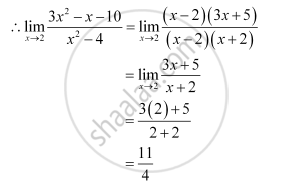CBSE (Arts) Class 11CBSE
Share

# Evaluate the Given Limit: Lim_(X- > 2) (3x^2 - X - 10)/(X^2- 4) - CBSE (Arts) Class 11 - Mathematics

ConceptIntuitive Idea of Derivatives

#### Question

Evaluate the Given limit: lim_(x- > 2) (3x^2 - x - 10)/(x^2- 4)

#### Solution

At x = 2, the value of the given rational function takes the form 0/0Is there an error in this question or solution?

#### APPEARS IN

NCERT Solution for Mathematics Textbook for Class 11 (2018 to Current)
Chapter 13: Limits and Derivatives
Q: 7 | Page no. 301
Solution Evaluate the Given Limit: Lim_(X- > 2) (3x^2 - X - 10)/(X^2- 4) Concept: Intuitive Idea of Derivatives.
S